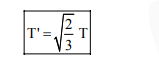# Time period of a simple pendulum is T inside a lift when the lift is stationary.

Question:

Time period of a simple pendulum is $\mathrm{T}$ inside a lift when the lift is stationary. If the lift moves upwards with an acceleration $\mathrm{g} / 2$, the time period of pendulum will be :

1. $\sqrt{3} \mathrm{~T}$

2. $\frac{T}{\sqrt{3}}$

3. $\sqrt{\frac{3}{2}} \mathrm{~T}$

4. $\sqrt{\frac{2}{3}} \mathrm{~T}$

Correct Option: , 4

Solution:

When lift is stationary

$\mathrm{T}=2 \pi \sqrt{\frac{\mathrm{L}}{\mathrm{g}}}$

When lift is moving upwards

$\Rightarrow$ Pseudo force acts downwards

$\Rightarrow \mathrm{g}_{\mathrm{eff}}=\mathrm{g}+\frac{\mathrm{g}}{2}=\frac{3 \mathrm{~g}}{2}$

$\Rightarrow$ New time period

$T^{\prime}=2 \pi \sqrt{\frac{\mathrm{L}}{\mathrm{g}_{\text {eff }}}}=2 \pi \sqrt{\frac{2 \mathrm{~L}}{3 \mathrm{~g}}}$